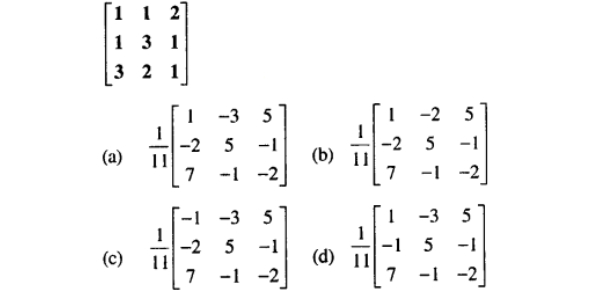# Mathematics MCQ: Quiz! Exam

20 Questions | Total Attempts: 470Settings.

• 1.
Solve the following inequality: 28 - 5x < x -4
• A.

X > 6

• B.

X < 5.33

• C.

X > 5.33

• D.

X > 8

• 2.
Which of the following is NOT a measure of central tendencies?
• A.

Median

• B.

Mean deviation

• C.

Decile

• D.

Mode

• 3.
What is the length of an arc, which subtends an angle 600 at the centre of a circle of radius 0.5m?
• A.

4.21

• B.

2.31

• C.

1.13

• D.

0.52

• 4.
Evaluate 7 - 35 = (mod 8).
• A.

-6

• B.

-4

• C.

2

• D.

4

• 5.
Esther was facing S20°W. She turned 90° in the clockwise direction. What direction is she facing now?
• A.

S70°E

• B.

N70°W

• C.

N70°E

• D.

S70°W

• 6.
What is the area of a circle whose radius is 7cm. (Take π = 227 ).
• A.

54cm2

• B.

84cm2

• C.

154cm2

• D.

184 cm2

• 7.
Find the circumference of a circle whose diameter is 10cm. (Take π = 227 ).
• A.

31.42cm

• B.

21.23cm

• C.

14.16cm

• D.

9.13 cm

• 8.
Write 123.6 in standard form.
• A.

1.236 X 10-1

• B.

1.236 X 101

• C.

1.236 X 102

• D.

1.236 X 103

• 9.
If y varies inversely as the square of x, and y = 1 1/4 when x = 4, find the value of y  when x= 1/2
• A.

- 1

• B.

5

• C.

10

• D.

15

• 10.
Use logarithm tables to evaluate the following: 608 x 4.85 ÷ 276.
• A.

10.69

• B.

9.69

• C.

8.96

• D.

1.06

• 11.
Three times a certain number added to 14 is the same as when two times the number is subtracted from 6. What is the number?
• A.

8/5

• B.

-8/5

• C.

-4

• D.

-8

• 12.
The angles of a pentagon are x0, 2x0, (x + 30)0, (x - 10)0 and (x + 40)0. Find x.
• A.

40°

• B.

60°

• C.

80°

• D.

120°

• 13.
Which of the following statements is true?
• A.

The angle of depression and the angle of elevation are the same angles in the same position

• B.

The angle of elevation and the angle of depression are different angles, but they have the same measure

• C.

The angle of depression and the angle of elevation have different measures

• D.

The angle of elevation and the angle of depression are both obtuse angles.

• 14.
A bicycle depreciates in value by about 15% each year. If Regina bought a bicycle for ₦6800 in 1988, find the value 3years later.
• A.

₦4176

• B.

₦6800

• C.

₦8176

• D.

₦20400

• 15.
Given the corner points of solution of inequation on some trading equipment are A (3 , -2), B (2 , 3), C (-5, 2), D (4 , 3). Minimize cost Z=3x+y.     (a) 7    (b) -9    (c) 13    (D) -13
• A.

7

• B.

-9

• C.

13

• D.

-13

• 16.
The mean of 2, 5, (x+1), (x+2), 7 and 9 is 6. Find the median
• A.

5.5

• B.

6.0

• C.

6.5

• D.

7.0

• 17.
The lengths of the adjacent sides of a right-angled triangle are x cm and (x-1) cm. If the length of the hypotenuse is √13, find the value of x.
• A.

2

• B.

3

• C.

4

• D.

5

• 18.
The sum to infinity of a geometric progression is -1/10 and the first term is  -1/8. Find the common ratio of the progression.
• A.

11/5

• B.

21/4

• C.

-1/3

• D.

-1/2

• 19.
Identify the invalid statement in the following: p:    A lazy boy shall not eat q:    Joseph is a leader and intelligent
• A.

• B.

• C.

P and q

• D.

P or q

• 20.
The common ratio of G.P. is 2. If the 5th term is greater than the first term by 45, find the 5th term.
• A.

45

• B.

46

• C.

47

• D.

48

Related TopicsBack to top